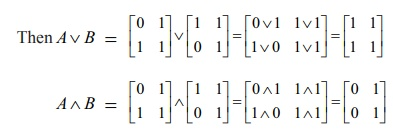Home | | Maths 12th Std | Some binary operations on Boolean Matrices

# Some binary operations on Boolean Matrices

A Boolean Matrix is a real matrix whose entries are either 0 or 1.

Some binary operations on Boolean Matrices

### Definition 12.3

A Boolean Matrix is a real matrix whose entries are either 0 or 1.

Note that the boolean entries 0 and 1 can be defined in several ways. In electrical switch to describe “on and off”, in graph theory, the “adjacency matrix” etc , the boolean entries 0 and 1 are used. We consider the same type of Boolean matrices in our discussion.

The following two kinds of operations on the collection of all boolean matrices are defined.

Let A = [aij] and B = [bij] be any two boolean matrices of the same type. Then their join and meet are defined as follows:

### Definition 12.4: Join of A and B

A B =  [aij] [bij] = [aij bij] = [cij]### Definition 12.5: Meet of A and B

A B = [aij] [bij] = [aij bij] = [cij]It is clear that ( a b) = max {a , b} ; ( a b) = min {a , b} , a , b {0, 1}.

### Example 12.8

Letbe any two boolean matrices of the same type. Find A B and AB.

### Solution## Properties satisfied by join and meet

Let B be the set of all boolean matrices of the same type. We only state the properties of meet and join.

### Closure property

A, B B, A B = [ aij ] [bij ] = [ aij bij ] B. (Because, ( aij bij ) is either 0 or 1 i , j . is a binary operation on B.

### Associative property

A(BC) = (A B) C, A,B,C B. is associative.

### Existence of identity property

A B, the null matrix 0  B A 0 = 0 A = A . The identity element for is the null matrix.

### Existence of inverse property

For any matrix A B, it is impossible to find a matrix

B B  ⋺A B = B A = 0 . So the inverse does not exist.

Similarly, it can be verified that the operation meet satisfies (i) closure property (ii) commutative property (iii) associative property (iv) the matrixexists as the identity in B and (v) the existence of inverse is not assured.

Tags : Discrete Mathematics | Mathematics , 12th Maths : UNIT 12 : Discrete Mathematics
Study Material, Lecturing Notes, Assignment, Reference, Wiki description explanation, brief detail
12th Maths : UNIT 12 : Discrete Mathematics : Some binary operations on Boolean Matrices | Discrete Mathematics | Mathematics